# MP Board Class 12th Maths Solutions Chapter 1 Relations and Functions Ex 1.2

In this article, we share MP Board Class 12th Maths Book Solutions Chapter 1 Relations and Functions Ex 1.2 Pdf, These solutions are solved by subject experts from the latest MP Board books.

## MP Board Class 12th Maths Solutions Chapter 1 Relations and Functions Ex 1.2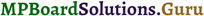Question 1.
Show that the function f : R* → R*, defined by f(x) = $$\frac { 1 }{ x }$$ is one-one and onto, where R* is the set of all non-zero real numbers. Is the result true, if the domain R* is replaced by N with co-domain being same as R*?
Solution:
a. One-one
Let x1, x1 ∈ R, such that f(x1) = f(x2)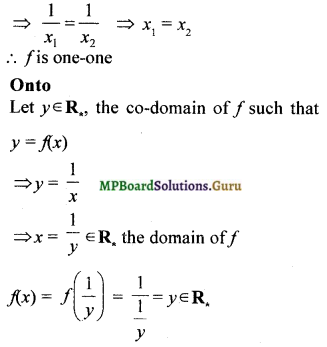For each y ∈ R* there exists x = $$\frac { 1 }{ y }$$ ∈ R,
such that f(x) = y.
∴ f is onto

b. One-one
Let x1, x2 ∈ N such that f(x1) = f(x2)
⇒ $$\frac{1}{x_{1}}=\frac{1}{x_{2}}$$ ⇒ x1 = x2
∴ f is one-one
Onto
The domain of f is N = {1, 2, 3, ……. }
The range of f = {1, $$\frac { 1 }{ 2 }$$, $$\frac { 1 }{ 3 }$$, $$\frac { 1 }{ 4 }$$, ……. } ≠ R
∴ f is not onto
Another method
2 ∈ R*.
The pre-image of 2 is $$\frac { 1 }{ 2 }$$ ∉ N .
Hence f is not onto.Question 2.
Check the injectivity and surjectivity of the following functions
i. f : N → N given by f (x) = x²
ii. f : Z → Z given by f (x) = x²
iii. f : R → R given by f (x) = x²
iv. f : N → N given by f (x) = x³
v. f : Z → Z given by f (x) = x³
Solution:
i. Injectivity
Let x1, x1 ∈ N such that f(x1) = f(x2)
⇒ x²1 = x²2
⇒ x²1 – x²2 = 0
⇒ (x1 – x2)(x1 + x2) = 0
⇒ x1 – x2 = 0 since x1 + x2 ≠ 0 as x1, x1 ∈ N
⇒ x1 = x2
∴ f is injective
Surjectivity
Let y = 3 ∈ N, the co-domain of f
f(x) = 3 ⇒ x² = 3
⇒ x = ±$$\sqrt{3}$$ ∉ N , the domain of f
∴f is not surjective

ii. Injectivity
Let x1 = 2, x2 = – 2
f(x1) = 2² = 4, f(x2) = (- 2)² = 4
i.e., f(x1) = f(x2) for x1 ≠ x2
∴ f is not injective

Surjectivity
Let y = 2 ∈ Z, the co-domain of f
∴ f(x) = 2 ⇒ x² = 2 ⇒ x = ± $$\sqrt{2}$$ ∉ Z, the domain of f
∴ f is not surjective.

iii. Injectivity
Let x1 = 2, x2 = – 2
f(x1) = 2² = 4, f(x2) = (- 2)² = 4
i.e., f(x1) = f(x2) for x1 ≠ x2
∴ f is not injective (one-one)

Surjectivity
Let y = – 1 ∈ R, the co-domain of f
f(x) = – 1 ⇒ x² = -1
⇒ x = ± $$\sqrt{1}$$ ∉ R, the domain of f
∴ f is not surjective (onto)

iv. Injectivity
Let x1, x2 ∈ N such that f(x1) = f(x1) = f(x2)
⇒ x³1 = x³2 ⇒ x³1 – x³2 = 0
⇒ (x1 – x2) (x²1 + x1x2 + x²22) = 0
⇒ x1 – x2 = 0 since x²1 + x1x2 + x²2 ≠ 0
⇒ x1 = x2
∴f is injective (one-one)

Surjectivity
Let y = 4 ∈ N, the co-domain of f
⇒ f(x) = 4 ⇒ x3 = 4
⇒ x = 41/3 ∉ N, the domain of f
∴f is not surjective (onto)

v. Injectivity
Let x1, x2 ∈ Z such that f(x1) = f(x2)
⇒ x³1 = x³2 ⇒ x1 = x2
∴ f is injective (one-one)

Surjectivity
Let y = 4 ∈ Z, the co-domain of f such that
f(x) = 4
⇒ x3 = 4 ⇒ x = 41/3 ∉ Z, the domain of f
∴ f is not surjective (onto)Question 3.
Prove that the Greatest Integer Function f : R → R, given by f(x) = [x], is neither one-one nor onto, where [x] denotes the greatest integer less than or equal to x.
Solution:
Refer Example 26
One-one
Let x1 = 2.1, x2 = 2.5
f(x1) = f(2.1) = [2.1] = 2
f(x2) = f(2.5) = [2.5] = 2
i.e. f(x1) = f(x2) for x1 ≠ x2
∴ f is not one-one.

Onto
Let y = 2.5 ∈ R, the co-domain of f
f(x) = 2.5 ⇒ [x] = 2.5, which is not possible.
∴ f is not onto

Question 4.
Show that the Modulus Function f : R → R, given by f(x) = |x|, is neither one-one nor onto, where |x| is x, if x is positive or 0 and |x| is – x, if x is negative.
Solution:
One-one
Let x1 = 1 and x2 = – 1 ∈ R
f(x1) = f(1) = |1| = 1
f(x2) = f(- 1) = |- 1|= 1
i.e., f(x1) = f(x2) for x1 ≠ x2
∴ f is not one-one Onto
Let y = – 1 ∈ R, the co-domain of f
f(x) = – 1 ⇒ |x|= – 1 which is not possible
∴ f is not onto.

Question 5.
Show that the Signum Function f : R → R, given by
$$f(x)=\left\{\begin{array}{c} 1, \text { if } x>0 \\ 0, \text { if } x=0 \\ -1, \text { if } x<0 \end{array}\right.$$
is neither one-one nor onto.
Solution:
One-one
Let x1 = 1, x2 = 2
f(x1) = f(1) = 1
f(x2) = f(2) = 1
i.e. f(x1) = f(x2) for x1 ≠ x2
∴ f is not one-one

Onto
The co-domain of f is R
The range of f is {-1, 0, 1} ≠ R
∴ f is not onto.Question 6.
Let A = {1, 2, 3}, B = (4, 5, 6, 7} and let f = {(1, 4), (2, 5), (3, 6)} be a function from A to B. Show that f is one-one.
Solution: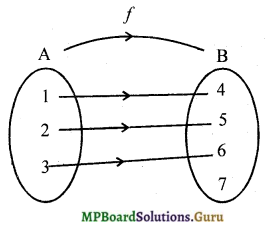Different elements in A have different images in B. Hence f is one – one.

Question 7.
In each of the following cases, state whether the function is one-one, onto or bijective. Justify your answer.
i. f : R → R defined by f(x) = 3 – 4x
ii. f : R → R defined by f(x) = 1 + x2
Solution:
i. One-one
Let x1, x2 6 R such that f(x1) = f(x2)
⇒ 3 – 4x1 = 3 – 4x2
⇒ – 4x1 = – 4x2
⇒ x1 = x2
∴f is one-one
Onto
Let y ∈ R, such that y = f(x)
⇒ y = 3 – 4x
⇒ 4x = 3 – y
⇒ x = $$\frac { 3-y }{ 4 }$$ ∈ R
f(x) = f$$\frac { 3-y }{ 4 }$$ = 3 – 4($$\frac { 3-y }{ 4 }$$)
= 3 – 3 + y = y
For each y ∈ R there exists x = $$\frac { 3-y }{ 4 }$$ ∈ R
such that f(x) = y
∴f is onto
Since f is one-one and onto, f is bijective.
Another Method
f(x) = 3 – 4x is a linear function from R to R.
∴ f is one-one and onto.
Hence f is bijective.

ii. One-one
Let x1 = 1, x2 = – 1 ∈ R
f(x1) = f(1) = 1 + 1² = 2
f(x2)= f(-1) = 1 + (- 1)² = 2
i.e., f(x1) = f(x2) for x1 ≠ x2
∴f is not one-one

Onto
Let y = – 1 ∈ R, the co-domain of f
f(x) = y ⇒ 1 + x2 = – 1
⇒ x2 = – 2
⇒ x = ± $$\sqrt{-2}$$ ∉ R
∴f is not onto.
Thus f is neither one-one nor onto.Question 8.
Let A and B be sets. Show that f : A x B → B x A such that f(a, b) = (b, a) is bijective function.
Solution:
Let (a1, b1) and (a2, b2) ∈ A x B
such that f(a1, b1) = f(a2, b2)
⇒ (b1, a1) = (b2, a2)
⇒ b1 = b2 and a1 = a2
⇒ (a1, b1) = (a2, b2)
∴f is one-one.
Let (b, a) ∈ B x A ⇒ b ∈ B and a ∈ A
⇒ (a, b) ∈ A x B
f(a, b) = (b, a)
i.e., corresponding to each (b, a) ∈ B x A, there exists (a, b) ∈ A x B such that f(a, b) = (b, a)
∴ f is onto
Hence f is a bijection.

Question 9.
Let f : N → N be defined by
f(n) = $$\left\{\begin{array}{l} \frac{n+1}{2}, \text { if } n \text { is odd } \\ \frac{n}{2}, \text { if } n \text { is even } \end{array} \text { for all } n \in \mathbf{N}\right.$$
Solution:
Let x1 = 1 and x2 = 2 ∈ N
f(x1) = f(1) = $$\frac { 1+1 }{ 2 }$$ = 1 and f(x2) = f(2) = $$\frac { 2 }{ 2 }$$ = 1
∴ f(x1) = f(x2) for x1 ≠ x2
Hence f is not one-one and hence not bijective.

Question 10.
Let A = R – {3} and B = R – {1}. Consider the function f : A → B defined by f(x) = $$\left(\frac{x-2}{x-3}\right)$$. Is f one-one and onto? Justify your answer.
Solution:
One-one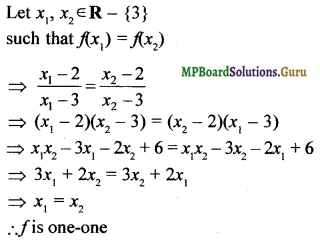One-one
Let y ∈ B such that f(x) = y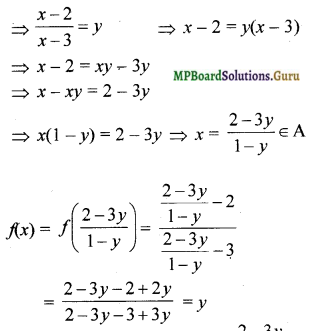For each y ∈ B, there exists x = $$\left(\frac{2-2y}{1-y}\right)$$ ∈ A
such that f(x) = y
∴ f is onto.Question 11.
Let f : R → R be defined as f(x) = x4. Choose the correct answer.
a. f is one-one onto
b. f is many-one onto
c. f is one-one but not onto
d. f is neither one-one nor onto.
Solution:
d. f is neither one-one nor onto.

One-one
Let x1 = 1, x2 = – 1 ∈ R
f(x1) = f(1) = (1)4 = 1
f(x2) = f(-1) = (-1)4= 1
f(x1) = f(x2) for x1 ≠ x2
∴ f is not one-one

Onto
The co-domain of f is R.
The range of f is [0, ∞), the non-negative real numbers.
Since range of f ≠ co-domain of f is not onto.
Hence f is neither one-one nor onto.

Question 12.
Let f : R → R be defined as f (x) = 3x. Choose the correct answer.
a. f is one-one onto
b. f is many-one onto
c. f is one-one but not onto
d. f is neither one-one nor onto.
Solution:
a. f is one-one onto
f(x1) = f(x2) ⇒ 3x1 = 3x2 ⇒ x1 = x2
∴ f is one-one
For y ∈ co-domain off there exists $$\frac { y }{ 3 }$$ in the domain of f such that f($$\frac { y }{ 3 }$$) – 3($$\frac { y }{ 3 }$$) = y
Hence f is onto.